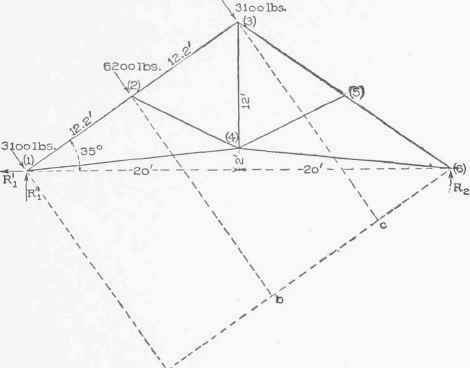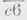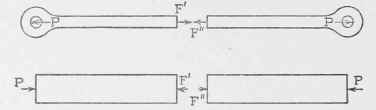"When the loads are not all vertical, the loads and the reactions constitute a non-concurrent non-parallel system and any one of the sets of conditions of equilibrium stated in Art. 35 may be used for determining the reactions. In general the fourth set is probably the simplest. In the first illustration we apply the four different sets for comparison.

Examples. 1. It is required to compute the reactions on the truss represented in Fig. 29 due to the wind pressures shown on the left side (3,100, 6,200and 3,100 pounds), the truss resting on rollers at the right end and being fastened to its support at the left.

(a) Let R1 and R2 denote the left and right reactions. The direction of R2 (at the roller end) is vertical, but the direction of R1 is unknown. Imagine R1 resolved into and replaced by its horizontal and vertical components and call them R1' and R1 respectively (see Fig. 35.) The six forces, R1, R1', R2 and the three wind pressures are in equilibrium, and we may apply any one of the sets of statements of equilibrium for this kind of a system (see Art. 35) to determine the reactions. If we choose to use the first set we find, resolving forces along a horizontal line,

-R1 + 3,100 cos 55° + 6,200 cos 55° + 3,100 cos 55° = 0; resolving forces along a vertical line,

+R1" + R2-3,100 cos 35°- 6,200 cos 35°- 3,100 cos 35° = 0 ; taking moments about the left end,

+ 6,200 X 12.2 + 3,100 X 24.4 - R2 X 40 = 0. From the first equation, R1'=3,100 cos 55°+ 6,200 cos 55° + 3,100 cos 55°=7,113 pounds, and from the third,

R2 = [,200 X 12.2 + 3,100 X 24.4 ] / 40 = 3,782 pounds.

Substituting this value of R2 in the second equation we find that

R, " =3,100 cos 35° + 6,200 cos 35° + 3,100 cos 35°- 3,782

= 10,156 - 3,782 = 6,374 pounds.Fig. 35.

If desired, the reaction R1 can now be found by compounding its two components R1' and R1".

(b) Using the second set of conditions of equilibrium stated in Art. 35 we obtain the following three "equilibrium equations " : As in (1), resolving forces along the horizontal gives

-R1'+ 3,100 cos 55° + 6,200 cos 55° + 3,100 cos 55° = 0, and taking moments about the left end,

6,200 X 12.2 + 3,100 X 24.4-R2X 40 = 0. Taking moments about the right end gives

R1' X 40 - 3,100 X- 6,200 X- 3,100 X=0

Just as in (a), we find from the first and second equations the values of R1' and R2. To find R1' we need values of the arms,, andBy measurement from a drawing we find that= 32.7,= 20. 5, and=8.3 feet.

Substituting these values in the third equation and solving for R1' we find that

R1" = [3,100x32.7+6,200x20.5+3,100x8.3] / 40=6,355 pounds

(c) Using the third set of conditions of equilibrium stated in Art. 35 we obtain the following three equilibrium equations : As in (b), taking moments about the right and left ends we get

R1" X 40-3,100 X 32.7-6,200 X 20.5-3,100 X 8.3 = 0, and -R2 X 40 + 6,200 X 12.2 + 3,100 X 24.4 = 0.

Choosing the peak of the truss as the origin of moments for the third equation we find that

R1' X 14 + R1" X 20 - 3,100 X 24.4 - 6,200 X 12.2 - R2 X 20 = 0.

As in (J) we find from the first two equations the values of R1' and R2. These values substituted in the third equation change it to

R1' X 14 + 6,373 X 20 - 3,100 X 24.4 - 6,200 X 12.2 - 3,782 X

20 = 0 or R 1 [ - 6.373 X 20 + 3,100 X 24.4 + 6,200 X 12.2 + 3,782 X 20]/14 = 7,104.*

(d) When using the fourth set of conditions we always determine the reaction at the roller end from the moment equation. Then, knowing the value of this reaction, draw the force polygon for all the loads and reactions and thus determine the magnitude and direction of the other reaction.

Taking moments about the left end, we find as in (a),(b), andFig. 36. Fig. 37.

(c) that E2 = 3,782. Then draw AB, BC and CD (Fig. 36) to represent the wind loads, and DE to represent R2. Since the force polygon for all the forces must close, EA represents the magnitude and direction of the left reaction; it scales 9,550 pounds,

2. It is required to determine the reactions on the truss of the preceding illustration when the wind blows from the right.

The methods employed in the preceding illustration might be used here, but we explain another which is very simple. The truss and its loads are represented in Fig. 37. Evidently the resultant of the three wind loads equals 12,400 pounds and acts in the same line with the 6,200-pound load. If we imagine this resultant to replace the three loads we may regard the truss acted upon by three forces, the 12,400-pound force and the reactions, and these three forces as in equilibrium. Now when three forces are in equilibrium they must be concurrent or parallel, and since the resultant load (12,400 pounds) and R2 intersect at O, the line of action of R1 must also pass through O. Hence the left reaction acts through the left support and O as shown. "We are now ready to determine the values of R1 and R2. Lay off AB to represent the resultant load, then from A and B draw lines parallel to R1 and R2, and mark their intersection C. Then BC and CA represent the magnitude and directions of R2 and R1 respectively; they scale 6,380 and 8,050 pounds.

* The slight differences in the answers obtained from the different sets of equilibrium equations are due to inaccuracies in the measured arms of some of the forces.Fig. 38.

## Example For Practice

1. Determine the reactions on the truss represented in Fig. 26 due to wind pressure, the distance between trusses being 15 feet, supposing that both ends of the truss are fastened to the supports.

 Ans. Reaction at windward end is 6,682½ pounds. Reaction at leeward end is 3,037½ pounds.